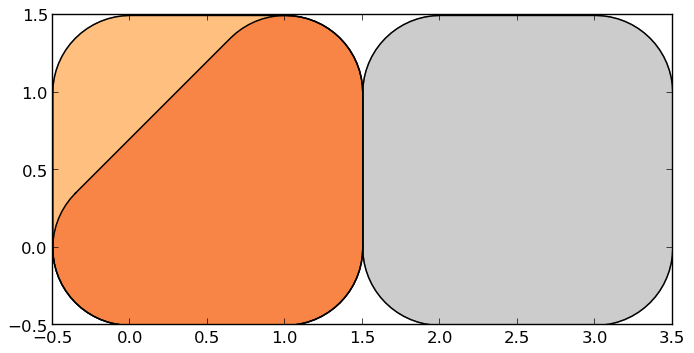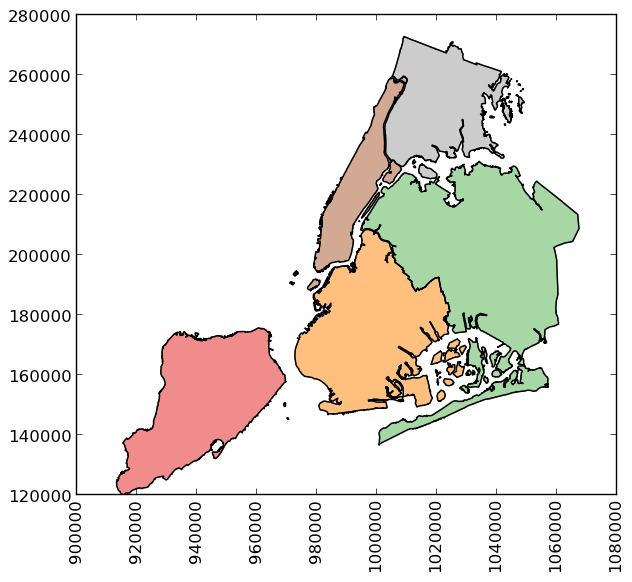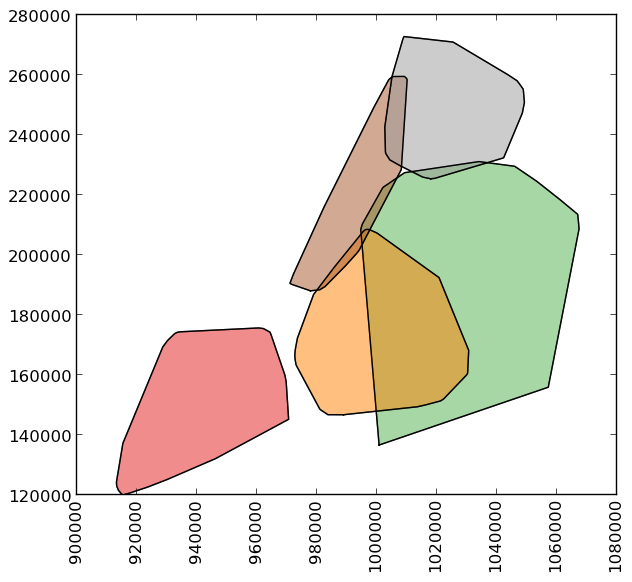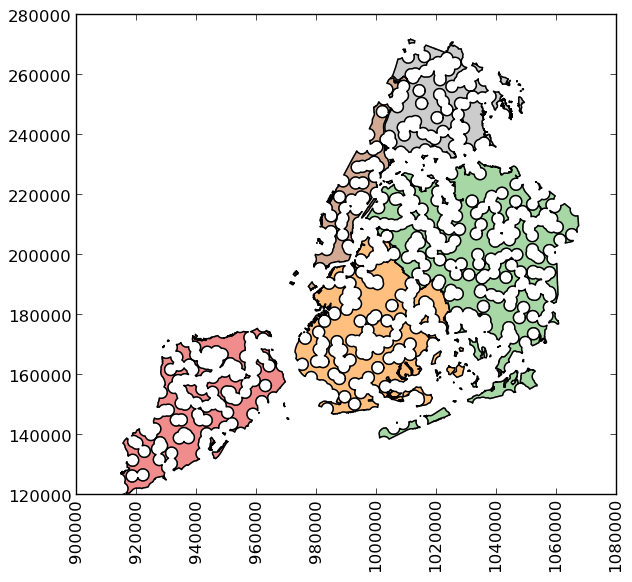# Geometric Manipulations¶

geopandas makes available all the tools for geometric manipulations in the *shapely* library.

Note that documentation for all set-theoretic tools for creating new shapes using the relationship between two different spatial datasets – like creating intersections, or differences – can be found on the set operations page.

## Constructive Methods¶

`GeoSeries.``buffer`(distance, resolution=16)

Returns a `GeoSeries` of geometries representing all points within a given distance of each geometric object.

`GeoSeries.``boundary`

Returns a `GeoSeries` of lower dimensional objects representing each geometries’s set-theoretic boundary.

`GeoSeries.``centroid`

Returns a `GeoSeries` of points for each geometric centroid.

`GeoSeries.``convex_hull`

Returns a `GeoSeries` of geometries representing the smallest convex Polygon containing all the points in each object unless the number of points in the object is less than three. For two points, the convex hull collapses to a LineString; for 1, a Point.

`GeoSeries.``envelope`

Returns a `GeoSeries` of geometries representing the point or smallest rectangular polygon (with sides parallel to the coordinate axes) that contains each object.

`GeoSeries.``simplify`(tolerance, preserve_topology=True)

Returns a `GeoSeries` containing a simplified representation of each object.

`GeoSeries.``unary_union`

Return a geometry containing the union of all geometries in the `GeoSeries`.

## Affine transformations¶

`GeoSeries.``rotate`(self, angle, origin='center', use_radians=False)

Rotate the coordinates of the GeoSeries.

`GeoSeries.``scale`(self, xfact=1.0, yfact=1.0, zfact=1.0, origin='center')

Scale the geometries of the GeoSeries along each (x, y, z) dimensio.

`GeoSeries.``skew`(self, angle, origin='center', use_radians=False)

Shear/Skew the geometries of the GeoSeries by angles along x and y dimensions.

`GeoSeries.``translate`(self, xoff=0.0, yoff=0.0, zoff=0.0)

Shift the coordinates of the GeoSeries.

### Examples of Geometric Manipulations¶

```>>> p1 = Polygon([(0, 0), (1, 0), (1, 1)])
>>> p2 = Polygon([(0, 0), (1, 0), (1, 1), (0, 1)])
>>> p3 = Polygon([(2, 0), (3, 0), (3, 1), (2, 1)])
>>> g = GeoSeries([p1, p2, p3])
>>> g
0    POLYGON ((0.0000000000000000 0.000000000000000...
1    POLYGON ((0.0000000000000000 0.000000000000000...
2    POLYGON ((2.0000000000000000 0.000000000000000...
dtype: object
```Some geographic operations return normal pandas object. The `area` property of a `GeoSeries` will return a `pandas.Series` containing the area of each item in the `GeoSeries`:

```>>> print g.area
0    0.5
1    1.0
2    1.0
dtype: float64
```

Other operations return GeoPandas objects:

```>>> g.buffer(0.5)
Out:
0    POLYGON ((-0.3535533905932737 0.35355339059327...
1    POLYGON ((-0.5000000000000000 0.00000000000000...
2    POLYGON ((1.5000000000000000 0.000000000000000...
dtype: object
```GeoPandas objects also know how to plot themselves. GeoPandas uses descartes to generate a matplotlib plot. To generate a plot of our GeoSeries, use:

```>>> g.plot()
```

GeoPandas also implements alternate constructors that can read any data format recognized by fiona. To read a zip file containing an ESRI shapefile with the borough boundaries of New York City (GeoPandas includes this as an example dataset):

```>>> nybb_path = geopandas.datasets.get_path('nybb')
>>> boros = GeoDataFrame.from_file(nybb_path)
>>> boros = boros.set_index('BoroCode')
>>> boros = boros.sort_index()
>>> boros
BoroName    Shape_Area     Shape_Leng  \
BoroCode
1             Manhattan  6.364422e+08  358532.956418
2                 Bronx  1.186804e+09  464517.890553
3              Brooklyn  1.959432e+09  726568.946340
4                Queens  3.049947e+09  861038.479299
5         Staten Island  1.623853e+09  330385.036974

geometry
BoroCode
1         (POLYGON ((981219.0557861328125000 188655.3157...
2         (POLYGON ((1012821.8057861328125000 229228.264...
3         (POLYGON ((1021176.4790039062500000 151374.796...
4         (POLYGON ((1029606.0765991210937500 156073.814...
5         (POLYGON ((970217.0223999023437500 145643.3322...
``````>>> boros['geometry'].convex_hull
0    POLYGON ((915517.6877458114176989 120121.88125...
1    POLYGON ((1000721.5317993164062500 136681.7761...
2    POLYGON ((988872.8212280273437500 146772.03179...
3    POLYGON ((977855.4451904296875000 188082.32238...
4    POLYGON ((1017949.9776000976562500 225426.8845...
dtype: object
```To demonstrate a more complex operation, we’ll generate a `GeoSeries` containing 2000 random points:

```>>> from shapely.geometry import Point
>>> xmin, xmax, ymin, ymax = 900000, 1080000, 120000, 280000
>>> xc = (xmax - xmin) * np.random.random(2000) + xmin
>>> yc = (ymax - ymin) * np.random.random(2000) + ymin
>>> pts = GeoSeries([Point(x, y) for x, y in zip(xc, yc)])
```

Now draw a circle with fixed radius around each point:

```>>> circles = pts.buffer(2000)
```

We can collapse these circles into a single shapely MultiPolygon geometry with

```>>> mp = circles.unary_union
```

To extract the part of this geometry contained in each borough, we can just use:

```>>> holes = boros['geometry'].intersection(mp)
```and to get the area outside of the holes:

```>>> boros_with_holes = boros['geometry'].difference(mp)
```Note that this can be simplified a bit, since `geometry` is available as an attribute on a `GeoDataFrame`, and the `intersection` and `difference` methods are implemented with the “&” and “-” operators, respectively. For example, the latter could have been expressed simply as `boros.geometry - mp`.

It’s easy to do things like calculate the fractional area in each borough that are in the holes:

```>>> holes.area / boros.geometry.area
BoroCode
1           0.602015
2           0.523457
3           0.585901
4           0.577020
5           0.559507
dtype: float64
```